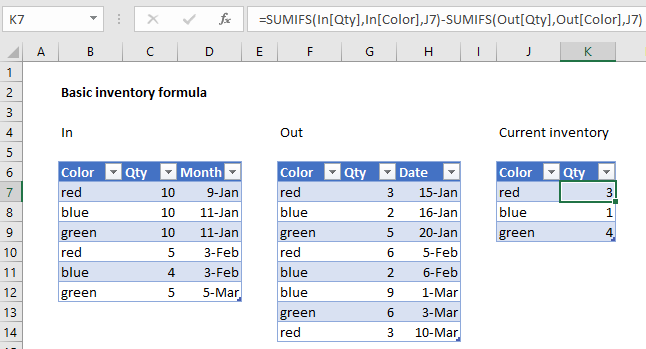## Excel Office

Excel How Tos, Tutorials, Tips & Tricks, Shortcuts

# How to calculate current stock or inventory in Excel

This tutorial covers basic inventory formula.

To calculate current stock, or inventory, you can use Excel Tables with a formula based on the SUMIF function.

## Formula

`=SUMIFS(In[Qty],In[Color],A1)-SUMIFS(Out[Qty],Out[Color],A1)`## Explanation

In the example shown, the formula in K7 is:

`=SUMIFS(In[Qty],In[Color],J7)-SUMIFS(Out[Qty],Out[Color],J7)`

Where “In” is the Excel Table on the left, “Out” is the table in the middle.

Worked Example:   Sum if equal to either x or y in Excel

### How this formula works

This formula demonstrates a very simple inventory concept where current inventory is simply the result of all incoming stock minus all outgoing stock. In the example, colors are treated as unique item identifiers – imagine a product available in one size only in just three colors: red, blue, or green.

Worked Example:   SUMIFS function: Description, Usage, Syntax, Examples and Explanation

The key to this approach is to use Excel Tables, because Table ranges automatically expand to handle changes in data. This means we can get a total of all incoming red items with:

`=SUMIFS(In[Qty],In[Color],J7)`

And a total of all outgoing red items with:

`=SUMIFS(Out[Qty],Out[Color],J7)`

In both cases, the SUMIFS function generates a total for all red items in each table.

Worked Example:   Sum if cells contain both x and y in Excel

Then, as long as both tables are up to date and complete, we can get the current inventory of red items with the following formula:

`=SUMIFS(In[Qty],In[Color],J7)-SUMIFS(Out[Qty],Out[Color],J7)`

As the formula is copied down, we get current inventory for each color.